# 数据结构（考纲总结）：八、内排序

14.667k 字  |  49 分钟

1. 排序的基本概念，各种内排序方法的基本原理和特点，包括排序过程中进行的元素之间的比较次数，排序总趟数、排序稳定性以及时间复杂度与空间复杂度计算；
2. 插入排序法（含折半插入排序法）；
3. 选择排序法；
4. （起）泡排序法；
5. 谢尔（Shell）排序法；
6. 快速排序法；
7. 堆积（Heap）排序法，包括堆积的定义与构造；
8. 各种排序方法的比较。

## 1. 排序的基本概念，各种内排序方法的基本原理和特点，包括排序过程中进行的元素之间的比较次数，排序总趟数、排序稳定性以及时间复杂度与空间复杂度计算；

### 1.1 排序的定义

$k_{\sigma(1)} \le k_{\sigma(2)} \le … \le k_{\sigma(n)}$

$k_{\sigma(1)} \ge k_{\sigma(2)} \ge … \ge k_{\sigma(n)}$

### 1.2 排序的分类

#### 1.2.1 内排序和外排序

1. 内部排序：当参加排序的数据量不大时，在排序过程中将全部信息存放在内存中处理的排序方法。
2. 外部排序：当参加排序的数据量较大时，以致于内存不足以一次存放全部信息，在排序过程中需要通过内存与外存之间的数据交换来达到排序目的，这种排序方式称为外排序。
• 外排序的速度要比内排序的速度慢很多。对于一些数据量较大的数据文件，由于计算机内存容量的限制，不能一次将全部数据装入内存进行排序，只能采用外排序来完成。

#### 1.2.2 稳定性排序与非稳定性排序

1. 稳定性排序：对于值相同的两个元素，排序前后的先后次序不变，则称该方法为 稳定性排序方法
2. 非稳定性排序：对于值相同的两个元素，排序前后的先后次序改变，则称该方法为 非稳定性排序方法

#### 1.2.3 连续顺序文件排序和链表排序

1. 连续顺序文件排序：由于记录之间的逻辑顺序是通过其物理地址的先后来映射，因而在排序过程中需要移动记录的位置。
2. 链表排序：文件中的一个记录对应着链表中的一个链结点，记录之间的逻辑顺序是通过指针来反应，因而排序过程中不必移动记录，只需修改相应指针的指向。

## 2. 插入排序法（含折半插入排序法）；

### 2.1 直接插入排序法（简单插入排序法）

#### 2.1.1 算法思想

• 第 i 趟排序将序列的第 i+1个元素 $k_{i+1}（i = 1，2，…，n-1）$ 插入到一个大小为 i，且已按值有序的序列 $k_1，k_2，…，k_i$ 的合适位置，得到一个大小为 i+1，并且仍然保持按值有序的序列 $k_1^"，k_2^"，…，k_{i+1}^"$ 。

#### 2.1.2 算法过程示例

• 例如有一个数据元素序列 $(49, 38, 65, 97, 76, 13, 27, 49)$，假设经过三趟排序以后，前 4 个元素已经按值从小到大次序排好序，即

$( \underline{38, 49, 65, 97}, 76, 13, 27, 49)$

• 现在要进行的第 4 趟排序把第 i = 5 个元素 76 插入到这个已经按值有序的子序列的合适位置，使得前 5 个元素组成的子序列仍然保持按值有序。那么，只需按照从后向前顺序查找的方法来确定元素 76 应该插入的位置。由于有 $65 \le 76 \le 97$，说明 76 应该插在 65 与 97 之间，也就是序列的第 4个位置。
• 这时，只要将序列中第 4 个位置到第 i-1 个位置上所有元素依次后移一个位置，然后再将元素 76 插入到第 4 个位置上即可。这样，又得到一个长度为 5 的新子序列，使得整个序列的前 5 个元素保持按值有序，即得到

$(\underline{38, 49, 65, 76, 97}, 13, 27, 49)$

• 此过程称为一趟插入排序。然后再按照上述原则分别将序列的第 6，7 个元素通过 3 趟排序依次将它们插入到其相应的合适位置，从而完成整个序列的排序。
• 在进行第 1 趟排序时，可以将序列的第 1 个元素看成一个长度为 1、且按值有序的子序列，然后只要依次从第 2 个元素开始逐个把其余 n-1 个元素插入到某个按值有序的子序列中就可以了。

#### 2.1.3 算法实现

void INSERT_SORT(int K[], int n) {
for (int i = 2; i <= n; i++) {
int temp = K[i]; /* 将当前被插入元素 K[i] 保存在 temp 中 */
int j = i-1;
while (j > 0 && temp < K[j]) {
K[j+1] = K[j]; /* 将比 K[i] 元素值大的元素后移 */
j--;
}
K[j+1] = temp; /* 插入到对应位置 */
}
}

#### 2.1.4 算法分析

• 对于具有 n 个元素的序列，插入排序方法一共要进行 n-1 趟排序。
• 对于插入排序算法，整个排序过程只需要一个辅助空间（temp）。
• 当原始序列是一个按值递增序列（升序）时，对应的每个 i 值只进行一次元素之间的比较，因而总的比较次数最少，为 $\sum_{i = 2}^n 1 = n - 1$，并不需要移动元素（记录），这是最好的情况。
• 最坏的情况是，序列初始时是一个按值递减序列（逆序），则对应的每个 i 值都要进行 i-1 次元素之间的比较，总的元素之间的比较次数达到最大值，为 $\sum_{i=2}^n (i-1) = n(n-1)/2$。
• 如果序列的初始情况是随机的，即参加排序的序列中元素可能出现的各种排列的概率相同，则可取上述最小值和最大值的平均值作为插入排序时所进行的元素之间的比较次数，约为 $n^2/4$。由此得知，插入排序算法的时间复杂度为 $O(n^2)$。
• 插入排序方法属于稳定性排序方法。

### 2.2 折半插入排序

#### 2.2.1 算法思想

• 由于每一趟被插入的子序列为一个按值有序的序列，因而可以采用折半查找方法来确定被插入元素在该有序序列中的合适位置。

#### 2.2.2 算法过程示例

• 例如，对于前面给出的序列 $(49, 38, 65, 97, 76, 13, 27, 49)$，采用折半插入排序法经过 6 趟排序以后，序列的前 7 个元素组成一个按值有序排列的序列，如下图所示是将序列的第 8 个元素 49 插入到这个按值有序序列中合适位置的第 7 趟的排序过程。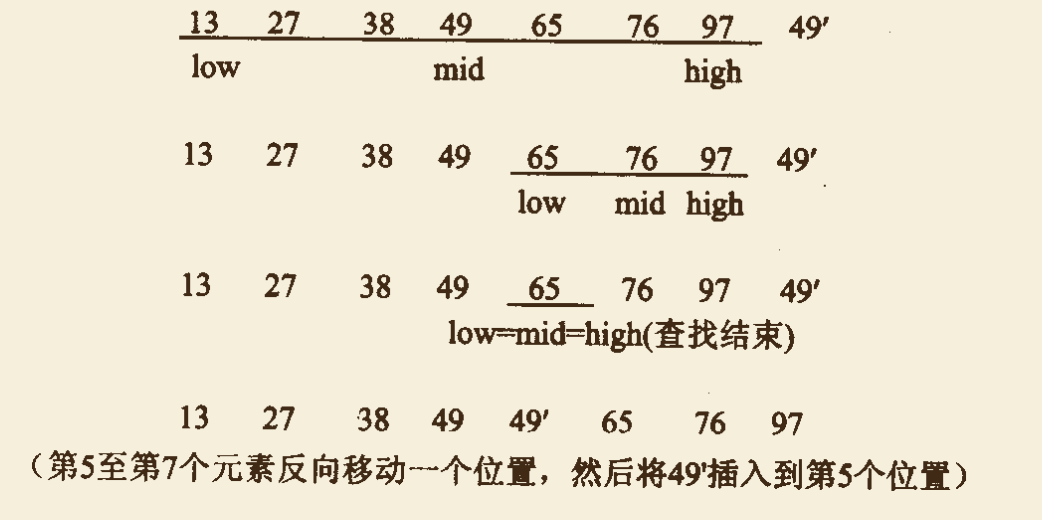#### 2.2.3 算法实现

void BIN_INSERT_SORT(int K[], int n) {
for (int i = 2; i <= n; i++) {
int temp = K[i]; /* 将当前被插入元素 K[i] 保存在 temp 中 */

int low = 1; /* 采用这半查找法确定 K[i] 的适合位置 */
int high = i-1;
while (low <= high) {
int mid = (low+high)/2;
if (temp < mid) {
high = mid - 1;
} else {
low = mid + 1;
}
}

for (int j = i-1; j >= low; j--) { /* 有关元素依次后移一个位置 */
K[j+1] = K[j];
}
K[low] = temp; /* 插入 K[i]，至此一趟结束 */
}
}

#### 2.2.4 算法分析

• 对于折半插入排序算法，依然需要一个辅助空间（temp）。
• 折半插入排序仅仅是减少了比较元素的次数，约为 $O(n log n)$，而且该比较次数与待排序表的初始状态无关，仅取决于表中的元素个数 n；而元素的移动次数没有改变，它依赖于待排序表的初始状态。因此，折半插入排序的时间复杂度仍然为 $O(n^2)$，但它的效果还是比直接插入排序要好。

## 3. 选择排序法；

### 3.1 算法思想

• 第 i 趟排序从序列的后 $n-i+1 (i = 1，2，…，n-1)$ 个元素中选择一个值最小的元素与该 $n-i+1$ 个元素的最前面那个元素交换位置，即与整个序列的第 i 个位置上的元素交换位置。如此下去，直到 $i = n-1$，排序结束。
• 简述为：每一趟排序从序列中未排好序的元素中选择一个值最小的元素，与未排好序的元素最前面的那个元素交换位置。

• 在算法中设置整形变量 i，既可以作为排序趟数的计算，同时也指出执行第 i 趟排序时，参加排序的后 $n-i+1$ 个元素的第 1 个元素的位置。
• 整形变量 mini 记录这 $n-i+1$ 个元素中值最小元素的位置。
• 每一趟排序开始，先另 $mini = i$ （即暂时假设序列的第 i 个元素为值最小者，以后经过比较后视实际情况再正式确定最小值元素的位置）。
• 第 i 趟排序比较结束时，这 $n-i+1$ 个元素中真正的值最小元素由此时的 mini 指出。此时，若有 $mini == i$，说明值最小元素就是这 $n-i+1$ 个元素的第 1 个元素，意味着此趟排序不必进行元素交换。

### 3.2 算法过程示例

• 例如，对于序列 $(49，38，65，97，76，13，27，49)$，第 1 趟排序从参加排序的全部元素中选择出值最小的元素 13（其位置由一个整形变量记录）。将 mini 位置上的元素 13 与第 1 个元素 49 交换位置后得到：$(\underline{13}，38，65，97，76，49，27，49)$。这就是第 1 趟 排序的结果。
• 第 2 趟排序就是在后面 7 个元素中再选择一个值最小的元素，并且将它与这 7 个元素的第 1 个元素交换位置，得到第 2 趟排序的结果。
• 以后每一趟排序都是这样。

i = 1 $(\underline{13}, 38, 65, 97, 76, 49, \overline{27}, 49)$
i = 2 $(\underline{13, 27}, 65, 97, 76, 49, \overline{38}, 49)$
i = 3 $(\underline{13, 27, 38}, 97, 76, \overline{49}, 65, 49)$
i = 4 $(\underline{13, 27, 38, 49}, 76, 97, 65, \overline{49})$
i = 5 $(\underline{13, 27, 38, 49, 49}, 97, \overline{65}, 76)$
i = 6 $(\underline{13, 27, 38, 49, 49, 65}, 97, \overline{76})$
i = 7 $(\underline{13, 27, 38, 49, 49, 65, 76}, \overline{97})$

### 3.3 算法实现

void SELECT_SORT(int K[], int n) {
for (int i = 1; i <= n-1; i++) {
int mini = i; /* 假设值最小元素为未排序元素的第 1 个元素 */
for (int j = i+1; j <= n; j++) {
if (K[j] < K[mini]) {
mini = j; /* 寻找真正值最小元素，记录其位置 mini */
}
}
if (mini != i) { /* 当值最小元素不是未排序元素的第 1 个元素时 */
int temp = K[i];
K[i] = K[mini];
K[mini] = temp; /* 值最小元素与未排序元素的第 1 个元素交换 */
}
}
}

### 3.4 算法分析

• 当原始序列是一个按值递增序列（升序）时，元素的移动次数最少，为 0 次。当序列初始时是一个按值递减序列（逆序）时，元素的移动次数最多，为 $3(n-1)$ 次（3 是交换 K[i] 与 K[mini] 的执行次数）。

• 但是，无论序列中元素的初始排列状态如何，第 i 趟排序要找出值最小元素都需要进行 $n - i$ 次元素之间的比较。因此，整个排序过程需要进行的元素之间的比较次数都相同，为 $\sum_{i = 2}^n (i-1) = n (n-1) / 2$ 次。

• 这说明选择排序法所进行的元素之间的比较次数与序列的原始状态无关，同时可以确定算法的时间复杂度为 $O(n^2)$。

• 由于值最小元素与未排好序的元素中第 1 个元素的交换动作是在不相邻的元素之间进行的，因此很有可能会改变值相同元素的前后位置，因此，选择排序法是一种不稳定的排序方法。

## 4. （起）泡排序法；

### 4.1 算法思想

• 先将序列中第 1 个元素与第 2 个元素进行比较，若前者大于后者，则两者交换位置，否则不交换；

• 然后将第 2 个元素与第 3 个元素比较，若前者大于后者，则两者交换位置，否则不交换；

• 依次类推，知道第 n-1 个元素与第 n 个元素比较（或交换）为止。经过如此一趟排序，使得 n 个元素中值最大元素被安置在序列的第 n 个位置上。

• 此后，再对前 n - 1 个元素进行同样过程，使得该 n - 1 个元素中值最大元素被安置在第 n - 1 个位置上。

• 然后再对前 n - 2 个元素重复上述过程，直到某一趟排序过程中不出现元素交换位置的动作，排序结束。

• 因此，（起）泡排序法核心思想可以简述为：第 i（i = 1，2，…）趟排序时从序列中前 n - i + 1 个元素的第 1 个元素开始，相邻两个元素进行比较，若前者大于后者，两者交换位置，否则不交换。

• （起）泡排序法是通过相邻元素之间的比较与交换，使值较小额元素逐步从后面移到前面，值较大的元素从前面移到后面，就像水底的气泡一样向上冒，故称次排序方法为冒泡排序法。

### 4.3 算法实现

• （起）泡排序过程中，元素之间进行比较的动作不可缺少，但元素之间是否交换位置却需要根据条件来确定。若某一趟排序过程中只有比较动作而无元素交换位置的动作，则说明这一趟排序位置序列已经按值有序，排序可以到此结束。
• 为了确定一趟排序中是否有交换动作，设置一个标志 flag，并约定：
$flag = \begin{cases} 1 & \text{说明某一趟排序过程中有元素交换的动作} \\ 0 & \text{说明某一趟排序过程中无元素交换的动作} \end{cases}$

每一趟排序之前，先将 flag 置 0，在排序过程中，只要出现元素交换位置的动作，就及时将 flag 置为 1。泡排序方法最多执行 n - 1 趟排序（当最小值元素处在原始序列的最后时），但至少要执行一趟排序（当原始序列为一个按值递增序列时）。

void BUBBLE_SORT(int K[], int n) {
int flag = 1;
for (int i = n-1; i >= 1; i--) {
if (flag == 0) {
break;
}

flag = 0; /* 每趟排序前标志 flag 置 0 */
for (int j = 1; j <= i; j++) {
if (K[j] > K[j+1]) {
int temp = K[j]; /* 交换两个元素的位置 */
K[j] = K[i];
K[i] = temp;
flag = 1; /* 标志 flag 置 1 */
}
}
}
}

### 4.4 算法分析

• 最好的情况下，初始时序列已经是从小到大有序（升序），则只需经过一趟 n - 1 次元素之间的比较，并且不移动元素，算法就可结束排序。此时，算法的时间复杂度为 $O(n)$。
• 最差的情况是当参加排序的初始序列为逆序，或者最小值元素处在序列的最后时，则需要进行 n - 1 趟排序，总共进行 $\sum_{i=2}^n (i-1) - n(n-1)/2$ 次元素之间的比较，因此，（起）泡排序算法的平均时间复杂度为 $O(n^2)$。
• 相比插入排序方法和选择排序方法，泡排序方法在排序过程中需要移动较多次数的元素。因此，跑排序方法比较适合于参加排序序列的数据量较小的情况，尤其是当序列的初始状态为基本有序的情况；而对于一般情况，这种方法是排序时间效率最低的一种方法。
• 由于元素交换是在相邻元素之间进行的，不会改变值相同元素的相对位置，因此，（起）泡排序法是一种稳定排序法。

## 5. 谢尔（Shell）排序法；

### 5.1 算法思想

• 首先确定一个元素间隔数 gap，然后将参加排序的序列按此间隔数从第 1 个元素开始一次分成若干个子序列，即分别将所有位置相隔为 gap 的元素视为一个子序列，在各个子序列中采用某种排序方法进行排序（例如，可用泡排序方法，也可利用其它排序方法）；然后减少间隔数，并重新将整个序列按新的间隔数分成若干个子序列，再分别对各个子序列进行排序，如此下去，直到间隔数 gap = 1。
• 谢尔排序法的主要特点是：由于排序的每一趟以不同的间隔数对子序列进行排序，因而元素的移动（小的元素往前移，大的元素往后移）在子序列之间跳跃式进行。gap 越大，跳跃的跨度就越大。很多情况下，当 gap = 1 时，序列几乎已经按值有序，不需要进行较多元素的移动就能达到排序目的。
• 谢尔排序算法利用了多重嵌套循环来实现，外循环是以各种不同的 gap 值对序列进行排序，直到 gap = 1。第 2 层循环是在某一个 gap 值下对各个子序列进行排序，若在某个 gap 值下发生了元素交换，则该循环还将继续，直到各子序列中均没有发生元素交换，这说明各子序列已经分别排好序。为了控制这一点，设置一个标志 flag，有：
$flag = \begin{cases}1 & \text{说明对各子序列排序时有元素交换的动作} \\ 0 & \text{说明对各子序列排序时没有元素交换的动作} \end{cases}$

在每一趟排序开始之前，首先将 flag 置 0；排序过程中若出现元素交换动作，将其置为 1。

• 对间隔数 gap 的取法也很多，实践证明，有一种比较常用的方法，它首先取 gap 为序列长度的一般，在排序过程中，后一趟排序 gap 值为前一趟排序 gap 值的一半，即：
\begin{align}gap_1 & = \lfloor n/2 \rfloor \\ gap_i & = \lfloor gap_{i-1}/2 \rfloor \quad i = 2，3，4，…\end{align}

其中，n 为序列中元素的总个数。

### 5.3 算法实现

void SHELL_SORT(int K[], int n) {
int gap = n;
int flag, temp;
while (gap > 1) {
gap /= 2;
do {
flag = 0; /* 每趟排序前,标志flag置0 */
for (int i = 1; i <= n-gap; i++) {
int j = i + gap;
if (K[i] > K[j]) {
temp = K[i];
K[i] = K[j];
K[j] = temp;
flag = 1;
}
}
} while (flag != 0);
}
}

### 5.4 算法分析

• 谢尔排序方法的速度是一系列间隔数 $gap_i$ 的函数，不太容易弄清楚比较次数与 gap之间的依赖关系，并给出完整的数学分析。
• 上面算法中，由于采用 $gap_i = \lfloor gap_{i-1}/2 \rfloor$ 的方法缩小间隔数，对于具有 n 个元素的序列，若 $gap_1 = \lfloor n/2 \rfloor$，则经过 $p = \lfloor log_2 n \rfloor$ 趟排序后就有 $gap_p = 1$，因此，谢尔排序方法的排序总躺数为 $\lfloor log_2 n \rfloor$。
• 从算法中也可以看到，最外层的 while 循环为 $log_2 n$ 数量级，中间层 do - while 循环为 $n$ 数量级。当子序列分得越多时，子序列内的元素就越少，最内层的 for 循环的次数也就越少；反之，当所分的子序列个数减少时，子序列内的元素也随之增多，但整个序列也逐步接近有序，而循环次数却不会随之增加。因此，谢尔排序算法的时间复杂度在 $O(n log_2 n)$ 与 $O(n^2)$ 之间。
• 谢尔排序方法是一种不稳定排序算法。

## 6. 快速排序法；

### 6.1 算法思想

• 从当前参加排序$(k_s，k_{s+1}，…，k_t)$ 中任选一个元素（通常称之为分界元素），把小于等于分界元素的所有元素都移到分界元素的前边，把大于等于分界元素的所有元素都移到分界元素后边。
• 这样，分界元素正好处在排序的最终位置上，并且将当前参加排序的元素分成前后两个子序列（前一个序列所有元素都小鱼等于分界元素，后一个子序列都大于等于分界元素）。
• 然后，分别对这两个子序列（若子序列长度大于 1）递归的进行上述过程，直到使得所有元素都到达整个排序后它们应处的最终位置上。

### 6.2 算法过程示例

• s：当前参加排序的那些元素的第一个元素在序列中的位置，初始值为 1。
• t：当前参加排序的那些元素的最后那个元素在序列中的位置，初始值为 n 。
• i，j : 两个位置变量，初始值分别为 s 与 t + 1。

1. 反复执行动作 $i ← i + 1$，直到 $K[s] \le K[i]$ 或者 $i = t$；然后反复执行动作 $j ← j − 1$，直到 $K[s] \ge K[j]$ 或者 $j = s$。
2. 若 $i < j$，则 $K[i]$ 与 $K[j]$ 交换位置，转到第1步。
3. 若 $i \ge j$，则 $K[s]$ 与 $K[j]$ 交换位置，到此，分界元素 $K[s]$ 的最终位置已经确定（位置为 j），然后对被 $K[s]$ 分成的两个子序列 $(k_s，…，k_{j-1})$ 和 $(k_{j+1}，…，k_t)$ 中长度大于 1 的部分重复上述过程，直到整个序列排序结束。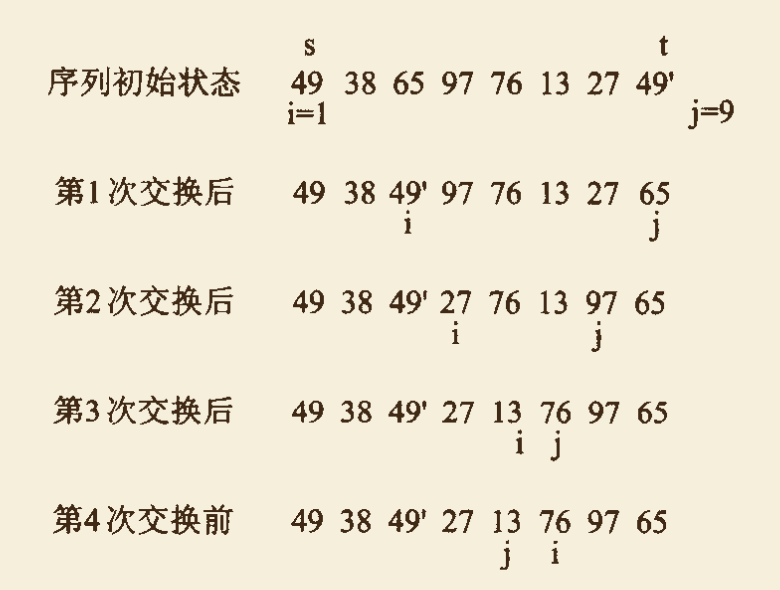• 从上图可以看出，第 4 次交换前出现 $j < i$ 的情况，按照算法思想，交换分界元素 $K[s]$ 与 $K[j]$ 两个元素的位置（第 4 次交换），得到 $(13, 38, 49^{'}, 27, 49, 76, 97, 65)$。

• 到此，分界元素 $K[s] = 49$ 初在序列的第 5 个位置，也就是它在整个排序结束后应处的最终位置，并且它将参加排序的这 8 个元素的序列划分为前后两个子序列 $(13, 38, 49^{'}, 27)$ 和 $(76, 97, 65)$。

• 前一个子序列中所有元素都不大于分界元素，后一个子序列中所有元素都不小于分界元素。由于这两个子序列的长度都超过 1，此时只需再分别对这两个子序列执行快速排序过程。

• 快速排序方法的每一次处理都可以确定分界元素排序的最终位置。实际上，很多情况下，快速排序方法一次处理可以确定多个元素（包括分界元素）的最终位置。

### 6.3 算法实现

void QUICK_SORT(int K[], int s, int t) {
if (s < t) {
int i = s;
int j = t+1;
while (1) {
do {
i++;
} while (!(K[s] <= K[i] || i == t));

do {
j--;
} while (!(K[s] >= K[j] || j == s));

if (i < j) { /* 交换 K[i] 和 K[j] 的位置 */
int temp = K[i];
K[i] = K[j];
K[j] = temp;
} else {
break;
}

}

int temp = K[s]; /* 交换 K[s] 和 K[j] 的位置 */
K[s] = K[j];
K[j] = temp;

/* 递归进行子序列的快速排序 */
QUICK_SORT(K, s, j-1);
QUICK_SORT(K, j+1, t);
}
}

### 6.4 算法分析

• 在参加排序的元素初始时已经有序的情况下，快速排序方法花费的时间最长。此时，第 1 趟排序经过 n - 1 次比较以后，将第 1 个元素仍然确定在原来的位置上，并得到 1 个长度为 n - 1 的子序列；第 2 趟排序进过 n-2 次比较以后，将第 2 个元素确定在它原来的位置上，又得到 1 个长度为 n - 2 的子序列；依次类推，最终总的比较次数为 $(n-1) + (n-2) + … + 1 = n(n-1)/2$。因此时间复杂度为 $O(n^2)$。
• 还有一种情况，若每趟排序后，分界元素正好定位在序列的中间，从而把当前参加排序的序列分成大小相等的前后两个子序列，则对长度为 n 的序列进行快速排序所需要的时间为：
\begin{align}T(n) \le & \ n + 2T(n/2) \\ \le & \ 2n + 4T(n/2) \\ \le & \ 3n + 8T(n/8) \\ & …… \\ \le & \ (log_2 n)n + nT(1) = O(nlog_2 n) \end{align}

因此，快速排序方法的时间复杂度为 $O(nlog_2 n)$，时间性能显然优于前面讨论的几种排序算法。

• 无论快速排序算法递归与否，排序过程中都需要用到堆栈或其他结构的辅助空间来存放当前待排序序列的首、尾位置。最坏的情况下，空间复杂度为 $O(n)$。
• 若对算法进行一些改写，在一趟排序之后比较被划分所得到的两个子序列的长度，并且首先对长度较短的子序列进行快速排序，这时候需要的空间复杂度可以达到 $O(log_2 n)$。
• 快速排序时一种不稳定的排序算法，也是一种不适合在链表结构上实现的排序算法。

## 7. 堆积（Heap）排序法，包括堆积的定义与构造；

### 7.1 堆积的定义

1. n 个元素的序列 $(k_1，k_2，…，k_n)$，当且仅当满足 $k_i \ge k_{2i}$ 并且 $k_i \ge k_{2i+1}$，$(i = 1，2，3，…， \lfloor n/2 \rfloor)$ 称该序列为一个 大顶堆积
2. n 个元素的序列 $(k_1，k_2，…，k_n)$，当且仅当满足 $k_i \le k_{2i}$ 并且 $k_i \le k_{2i+1}$，$(i = 1，2，3，…， \lfloor n/2 \rfloor)$ 称该序列为一个 小顶堆积
3. 堆积是一棵完全二叉树，二叉树中任何一个分支结点的值都大于或者等于它的孩子结点的值，并且每一棵子树也满足堆积的特性。

### 7.2 算法思想

• 首先设法将原始序列构造成第 1 个大顶堆（初始堆积），使得 n 个元素的最大值处于序列的第 1 个位置；
• 然后交换序列的第 1 个元素（最大值元素）与最后一个元素的位置。
• 此后，再把序列的前 n-1 个元素组成的子序列设法构成一个新的大顶堆，这样又得到第 2 个最大值元素，把子序列的第 1 个元素（最大值元素）与第 n - 1 个元素交换位置。
• 此后再把序列的前 n - 2 个元素再构成一个新的大顶堆，……，依次下去，最终把整个序列变换成一个按值有序的序列。
• 简单地说：堆积排序方法的第 i 趟排序就是将序列的前 n-i+1 个元素组成的子序列转换为一个堆积，然后将堆积的第 1 个元素与堆积的最后那个元素交换位置。

1. 建立初始堆积。
2. 交换堆积的第 1 个元素（最大值元素）与堆积的最后那个元素的位置。
3. 将移走最大值元素之后的剩余元素组成的序列再转换为一个堆积。
4. 重复上述过程的第 2 步和第 3 步 n - 1 次。

1. 将序列构造为初始堆积。
2. 如何将移走最大值元素之后的剩余元素组成的序列再转换为一个堆积。

### 7.3 堆积调整方法

#### 7.3.1 算法描述

• 下图（a）给出了由 n = 9 个元素组成的序列所对应的初始堆积。当第 1 个元素与第 9 个元素交换位置以后，前 8 个元素组成的序列所对应的完全二叉树如图（b）所示。
• 可以看出，这颗二叉树不是堆积。但是除了根结点以外，其余任何一棵子树仍然满足堆积的特性。
• 我们只需从根结点开始，自上而下地调整结点的位置，使其成为堆积。即把序号为 i 的结点与其左、右子树根结点（序号分别为 $2i$、$2i+1$）中值最大者及交换位置。如图（b）所示的完全二叉树，根结点 18 与其左、右子树的根结点最大者（即 41）交换位置以后得到图（c）所示的状态。
• 由于交换了位置，是的根结点右子树原有的堆积特性被破坏。于是，又要从这课右子树的根结点开始继续进行类似的调整。
• 如此下去，直到整棵完全二叉树成为一个堆积，如图（d）。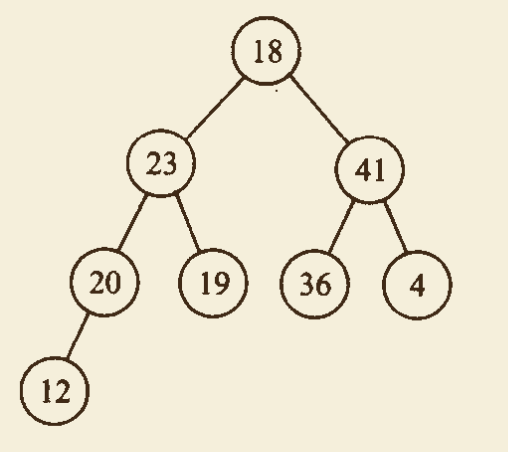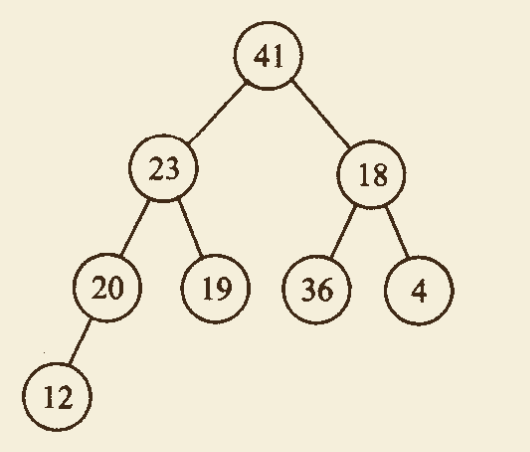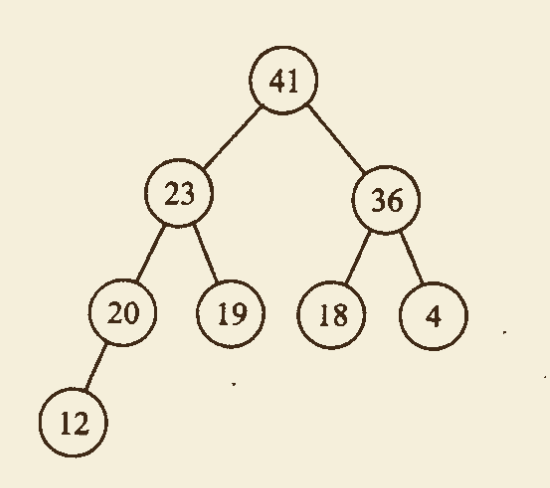• 完成 $k_i$ 与其左、右子树的根结点 $k_{2i}$ 和 $k_{2i+1}$ 中最大值者交换位置；
• 若交换位置以后破坏了子树的堆积特性，则再对这棵子树重复这个交换位置的过程，直到以结点 i 为根结点的子树成为堆积。

#### 7.3.2 算法实现

/**
* K[]: 序列
* i: 被调整的二叉树的根结点序号
* n: 被调整的二叉树结点数目
*/
void ADJUST(int K[], int i, int n) {
int temp = K[i]; // temp 存放根结点 i 的值
int child = 2*i; // child 为 i 结点左孩子序号
while (child <= n) {
if (child < n && K[child] < K[child+1]) { // child 给出 i 结点左、右孩子中值最大者的序号
child++;
}

if (temp >= K[child]) { // 如果根结点比左、右孩子中值最大者 还要大，则跳出循环。
break;
}
K[child/2] = K[child]; // 把左、右孩子中值最大者结点上移到父结点位置
child *= 2;
}
K[child/2] = temp; // 将根结点 i 的值放到最终位置
}

### 7.4 初始堆积建立方法

#### 7.4.1 算法描述

• 若原始序列对应的完全二叉树（不一定是堆积）的深度为 d，则从第 d - 1 层最右边的那个分支结点（器序号为 $\lfloor n/2 \rfloor$）开始，即初始时令 $i = \lfloor n/2 \rfloor$，调用算法 $ADJUST(K, i, n)$。

• 每调用一次该算法，执行一次 $i ← i + 1$ 的动作，直到 $i = 1$ 时，再调用一次，就能把原始序列调整为一个初始堆积。

• 在建立初始堆积之后，移走最大值元素，剩余元素组成的序列中只有序号 1 的元素破坏了整个二叉树堆积的特性，因此，每一趟排序只需对剩余元素组成的序列调用一次 $ADJUST(K, 1, i)$，便可以得到一个新的堆积。

#### 7.4.2 算法实现（完整的堆排序算法，包含初始堆积建立方法）

void HEAP_SORT(int K[], int n) {
// 构建初始顶堆
for (int i = n/2; i >= 1; i--) {
// 从第一个非叶子结点从下至上，从右至左调整结构
}
// 交换堆顶元素与末尾元素，调整堆结构
for (int i = n-1; i >= 1; i--) {
int temp = K[i+1]; // 将堆顶元素与末尾元素进行交换
K[i+1] = K;
K = temp;
}

for (int i = 1; i <= n; i++) {
printf(" %d",K[i]);
}
printf("\n");
}

### 7.6 算法分析

• 堆积排序的时间主要花费在将原始序列调整为一个初始堆积以及排序过程中不断将移走最大值元素以后剩下的那些元素重新调整为一个新堆积这两个部分上。因此，堆积方法对于 n 较大的数据元素序列是是很合适的。
• 设原始序列所对应的完全二叉树深度为 d，算法由两个独立的循环组成：
• 在第 1 个循环构造初始堆积时，从 $i = d-1$ 层开始，到 $i = 1$ 层为止，对每个分支节点都要调用一次 $ADJUST$ 算法，每一次 $ADJUST$ 算法，对于第 $i$ 层一个结点到第 $d$ 层上建立的子堆积，所有结点可能移动的最大距离为该子堆积根结点移动到最后一层（第 d 层） 的距离即 $(d-i)$。
• 而第 i 层上节点最多有 $2^{i-1}$ 个，所以每一次 $ADJUST$ 算法最大移动距离为 $2^{i-1} * (d-i)$。因此，堆积排序算法的第 1 个循环所需时间应该是各层上的结点数与该层上结点可移动的最大距离之积的总和，即：
$\sum_{i = d - 1}^1 2^{i-1} (d-i) = \sum_{j = 1}^{d-1} 2^{d-j-1} \times j = \sum_{j = 1}^{d-1} 2^{d-1} \times {j \over 2^j} \le n \sum_{j = 1}^{d-1} {j \over 2^j} < 2n$
这一部分时间花费为 $O(n)$。
• 在算法的第 2 个循环中每次调用 $ADJUST$ 算法一次，结点移动的最大距离为这棵完全二叉树的深度 $d = \lfloor log_2(n) \rfloor + 1$，一共调用了 $(n-1)$ 次 $ADJUST$ 算法，所以，第 2 个循环的时间花费为 $(n-1)(\lfloor log_2 (n)\rfloor + 1) = O(n log_2 n)$。
• 因此，堆积排序的时间复杂度为 $O(nlog_2 n)$。
• 由于在堆积排序中只需要一个记录大小的辅助空间，因此，堆积排序的空间复杂度为：$O(1)$。
• 堆积排序也是一种不适合在链表上实现的排序。
• 堆积排序属于不稳定排序算法。

## 8. 各种排序方法的比较

### 8.1 稳定性比较

• 稳定排序方法：插入排序、（起）泡排序、二路归并排序、基数排序方法
• 不稳定排序方法：选择排序、谢尔排序、快速排序、堆积排序方法

### 8.2 复杂度比较

（起）泡排序 $O(n^2)$ $O(n^2)$ $O(1)$

# 评论（没有评论）# 程序员充电站微信支付支付宝